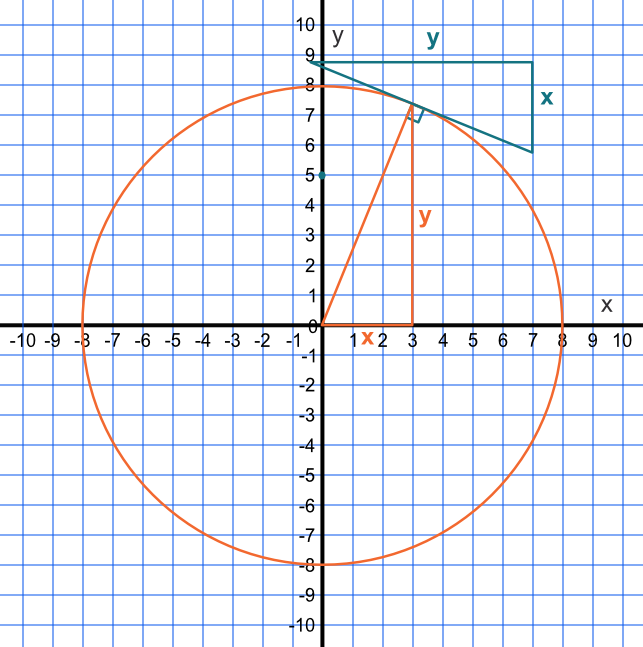Tangent to the Graph of a Circle

## Tangent to the Graph of a Circle

If a circle has a radius with centre at the origin, then a point on the circle can be described as being at a point (x, y). The gradient of the line to that point is frac(y)(x).

A line perpendicular to that radius is given by frac(-1)(text(gradient)) (see perpendicular lines). If the original gradient is frac(y)(x), then the gradient of the perpendicular line is frac(-x)(y).## Example 1

A circular function, centred on the origin, has a radius of 5.

What is the gradient of the tangent to the circle when x = 4?

The y-coordinate can be found by solving r^2 = x^2 + y^2

substituting 5^2 = 4^2 + y^2

25 = 16 + y^2, giving y = 3

The gradient of the radius at (4, 3) is frac(3)(4). Use the inverse of gradient, and multiply by -1: the tangent is -frac(4)(3).

Answer: -frac(4)(3)

## Example 2

The gradient of a tangent to a circle is -frac(7)(4). The circle is centred on the origin. If the radius of the circle is sqrt(585), what are the coordinates of the tangent?

Gradient of the radius to the tangent is the negative inverse of -frac(7)(4) = frac(4)(7). The radius of a circle is given by r^2 = x^2 + y^2

In this instance the radius is a multiple:

(mx)^2 + (my)^2 = (mr)^2

(7m)^2 + (4m)^2 = (sqrt(585))^2

49m^2 + 16m^2 = 585

65m^2 = 585, therefore m = 3

The tangent is therefore at (3 x 7, 3 x 4) = (21, 12)